# KSEEB Solutions for Class 9 Maths Chapter 3 Lines and Angles Ex 3.2

In this chapter, we provide KSEEB SSLC Class 9 Maths Chapter 3 Lines and Angles Ex 3.2 for English medium students, Which will very helpful for every student in their exams. Students can download the latest KSEEB SSLC Class 9 Maths Chapter 3 Lines and Angles Ex 3.2 pdf, free KSEEB SSLC Class 9 Maths Chapter 3 Lines and Angles Ex 3.2 pdf download. Now you will get step by step solution to each question.

## Karnataka Board Class 9 Maths Chapter 3 Lines and Angles Ex 3.2

Question 1.
In Fig. 3.28. find the values of x and y and then shows that AB||CD.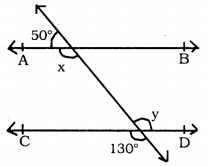i) ∠x = ?
ii) ∠y = ?
iii) Show tht AB||CD.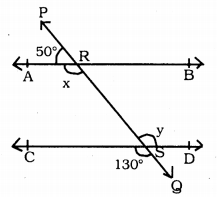(i) PQ Bisects AB at R.
∠PRA and ∠ARS are on straight line PQ.
∴ ∠PRA + ∠ARS = 180° Adjacent angles
50 + ∠ARS = 180°
∠ARS = 180 – 50
∴ x = ∠ARS = 130° (i)

(ii) Similarly, PQ straight line intersects straight line CD at S.
∴ ∠CSQ = ∠RSD . Vertically opposite angles
∴ y = ∠RSD = 130° (ii)
From (i) and (ii),
∠ARS = ∠RYD
x = y = 130°

(iii) Therse are mutual alternate angles
∴ x = y = 130°
∴ AB || CD.

Question 2.
In Fig. 3.29, if AB || CD, CD || EF and y : z = 3 : 7, find x.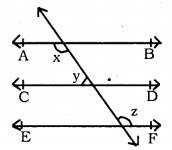PQ straight line intersects AB||CD]|EF at points R, S, T.
If y : z = 3 : 7, then value of ‘x’ = ?
PQ straight line intersects CD||EF at S and T.
∴ ∠CSR = ∠DST = y (∵ vertically opposite angle)
But, ∠DST + ∠STF = 180°
(∵ Sum of interior angles in the same side)
∴ y + z = 180°
But, y : z = 3 : 7.
Sum of ratio 3 + 7 = 10
If ratio 10 means 180
If ratio 9 means ?
180×310=54∘
∴ y = 54°
y + z = 180
54 + z = 180
∴ z = 180 – 54
∴ z = 126°
But, x + y = 180
(∵ Sum of interior angles on one side)
x + 54 = 180
∵ x = 180 – 54
∵ x = 126°.

Question 3.
In Fig. 3.30, If AB||CD, EF ⊥ CD and ∠GED = 126°, find ∠AGE, ∠GEF and ∠FGE.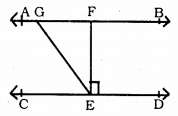AB||CD and EF⊥CD and ∠GED = 126°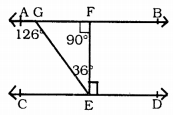∠AGE =?
∠GEF =?
∠FGE =?
∠GED = 126°.
∴ ∠GEF + ∠ZFED = 126°
∠GEF + 90° = 126° ∵ EF ⊥ CD.
∴ GEF = 126-90
∴  GEF = 36°.
∴ ∠GFE = 90° . EF ⊥ AB
∴ In ∆GEF
∠FGE + ∠GFE + ∠GEF = 180°
∠FGE + 90° + 36° = 180°
∠FGE + 126° = 180°
∴ ∠FGE = 180-126
∴ ∠FGE = 54°.
∠AGE + ∠FGE = 180° (Adjacent angles)
∠AGE + 54° = 180°
∴ ∠AGE =180-54
∠AGE = 126°
∴ ∠AGE = 126°
∠GEF = 36°
∠FGE = 54°.

Question 4.
In Fig. 3.31, If PQ||ST, ∠PQR =110°, and ∠RST = 130°, find ∠QRS.
(Hint: Draw a line parallel to ST through point R).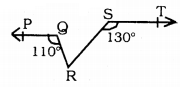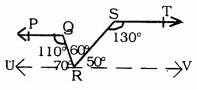Data: PQ||ST and ∠PQR= 110° and ∠RST= 130°.
To Prove: ∠QRS =?
Construction: Draw ST||UV through ‘R’
Proof: PQ||ST (Data)
ST || UV (Construction)
∴ PQ||ST||UV
PQ||UV.
∴ ∠PQR + ∠URQ = 180° (Sum of interior angles)
110 + ∠URQ = 180°
∠URQ = 180- 110
∴ ∠URQ = 70° (i)
Similarly, ST||UV.
∴ ∠RST + ∠SRV = 180°
130 + ∠SRV = 180
∴ ∠SRV = 180 – 130
∴ ∠SRV = 50° (ii)
But, URV is a straight line.
∴ ∠URV = 180°.
∠URQ + ∠QRS + ∠RSV = 180°
70 + ∠QRS + 50 = 180
∠QRS + 120 = 180°
∴ ∠QRS = 180 – 120
∴ ∠QRS = 60°.

Question 5.
In Fig. 3.32. if AB||CD, ∠APQ = 50° and ∠PRD = 127°, find x and y.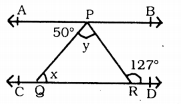If AB || CD and ∠APQ= 50° and ∠PRD = 127°, then ∠x = ? ∠y = ?
AB || CD PQ straight line intersects these at P and Q.
∴ ∠APQ = ∠PQR . alternate angles.
50 = ∠PQR = x
∴ x = 50°
Similarly, AB || CD PR straight line intersects these at P and R.
∴ ∠APR = ∠PRD
∠APQ + ∠QPR = ∠PRD
50 + ∠QPR = 127
∠QPR = 127 – 50
∴∠QPR = 77 °
∠QPR = y = 77 °
∴ x = 50 °,
y = 77 °.

Question 6.
In Fig. 3.33, PQ and RS are two mirrors placed parallel to each other. An incident ray AB strikes the mirror PQ at B, the reflected ray moves along the path BC and strikes the mirror RS at C and again reflects back along CD.
Prove that AB||CD.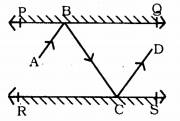Data: PQ and RS are two mirrors placed parallel to each other. An incident ray AB strikes the mirror PQ at B. the reflected ray moves along the path BC and strikes the mirror RS at C and again reflects back along CD.
To Prove: AB || CD.
Proof: PQ is a mirror.
AB is an incident ray, BC is a reflected ray. ∠ABC is the angle of incidence.
∠BCD is an angle of reflection.
The incident ray formed in the PQ mirror is equal to the reflected ray formed in the RS mirror.
∴ ∠ABC = ∠BCD.
These are pair of alternate interior angles, then AB || CD.

All Chapter KSEEB Solutions For Class 9 Maths

—————————————————————————–

All Subject KSEEB Solutions For Class 9

*************************************************

I think you got complete solutions for this chapter. If You have any queries regarding this chapter, please comment on the below section our subject teacher will answer you. We tried our best to give complete solutions so you got good marks in your exam.

If these solutions have helped you, you can also share kseebsolutionsfor.com to your friends.

Best of Luck!!